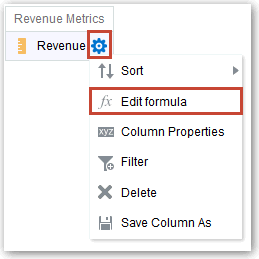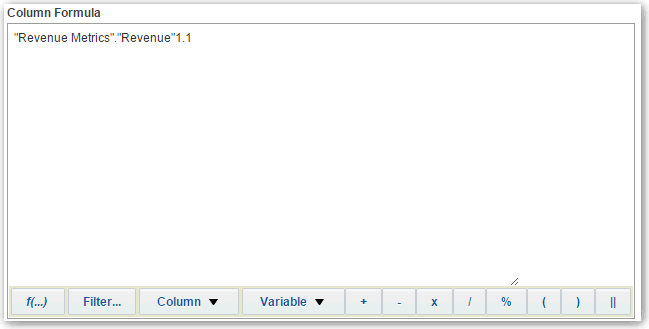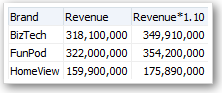Edit Formulas or Calculated Measures

You can fine-tune the columns in an analysis by editing the formulas of columns or editing calculated measures.

Topics:

Edit the Formula for a Column

You can edit the formulas for attribute columns and measure columns when you specify the criteria for an analysis. This editing affects the column only in the context of the analysis and doesn’t modify the formula of the original column in the subject area.

A column formula specifies what the column values represent. In its most basic form, such as "Revenue Metrics"."Revenue", a column takes the data from the data source as is. You can edit the formula to add functions, conditional expressions, and so on. This editing enables you to present analysis results in a variety of ways. For example, you can edit the formula of a Revenue column to display values after a 10% increase in revenue. You can do this by writing a formula that multiplies the Revenue column by 1.1.

1. Open the analysis for editing.
2. In the Selected Columns pane, click Options beside the column name, and then select Edit Formula.Description of the illustration analysis82.gif
3. On the Column Formula tab of the Edit Column Formula dialog, enter a formula in the Column Formula pane.Description of the illustration analysis29.gif

By default, the name of the column on the Selected Columns pane is displayed in the Column Formula pane.

• Use the operator and character buttons on the bottom of the Column Formula pane to help build the formula.

• Use the f(...) button to display the Insert Function dialog that enables you to include a function in the column formula. For example, you can build a formula based on a SQL function, such as RANK("Sales Measures"."Dollars"). See Expression Editor Reference.

• Use the Filter... button to display the Insert Filter dialog that enables you to include a filter expression in the column formula. Start the filter expression with at least one measure column. Include a Boolean expression that contains no measure columns or nested queries.

For example, you can build a formula that uses the SQL FILTER function to filter the data, such as FILTER("Sales Measures"."Dollars" USING ("Markets"."Region" = 'EASTERN REGION').

• Reference a column name in the formula using the form Folder-Name.Column.Name. If either the folder name or the column name includes non-alphanumeric characters (such as spaces or underscores), then enclose each name in double quotes. You can enclose the names in double quotes even if they have all alphanumeric characters.

• Use single quotes to include literals or constants that have a data type of string. For example, you can include constants such as ‘John Doe’ or ‘Best Selling Product’ in a formula.

4. Click OK. On the Results tab, the column displays its values with the formula applied.Description of the illustration analysis30.gif

Edit Calculated Measures

You can use calculated measures that are derived from other measures and created by using formulas.

For example, you might need to find the value after discounts are taken off the revenue. You create a calculated measure that subtracts the Discounted Amount from the Revenue value.

1. Open the analysis for editing.
2. On the Results tab, click New Calculated Measure on the toolbar to display the dialog.
3. If you have administrator privileges and want to customize folder and column headings with HTML markup, including JavaScript, select Contains HTML Markup, and then enter the HTML markup you want to apply. For examples, see Advanced Techniques: Format with HTML Tags.
4. Edit the formula for the calculated measure.
5. Click OK.